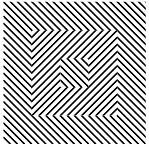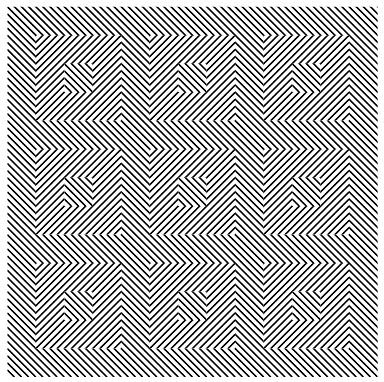OpArt is, by definition, a style of visual art based upon optical illusions. Let it be a painting, a photograph or any other mean, the objective of this style is to play with the interaction of what you see, and what it really is. A classical OpArt piece involves confusion by giving impression of movement, impossible solids, hidden images, conflicting patterns, warping, etc. And of course, Mathematics is a perfect vehicle to study—and even perform—this form of art.In this post I would like to show an example of how to use trivial mathematics to implement a well-known example (shown above) in $$\LaTeX$$ with the tikz package, and leave as homework another more interesting example.

Observe first the image above: the optical effect arises when conflicting concentric squares change the direction of their patterns. You may think that the color is the culprit of this effect but, as you will see below, it is only the relationship between the pure black-and-white patterns what produces the impression of movement.

Let us start by creating squares with both patterns. Notice that there is actually a single pattern, which is rotated 90º:

In both cases, we coded the square as a function of three variables: the size, and the location (as a point $$(x,y)$$ in the canvas). For example, (inside of a tikzpicture environment) the command \squareL{5}{0}{0}</tt> places a square with one of the patterns, and this square will have corners at the origin and the points $$(0,5), (5,0)$$ and $$(5,5)$$.Notice how this pattern alone starts producing that optical effect by itself. Let us change the pattern in consecutive steps, including two more concentric squares inside of this one, like so:For a stronger effect, combine several of these in a bigger image, taking advantage of the \foreach` command:The second example is a little bit more involved, but I am sure the reader will find the challenge interesting enough to give it a try. How would you code the following piece of OpArt? (in $$\LaTeX$$ or otherwise)Movement in Squares, by Bridget Riley (1961)